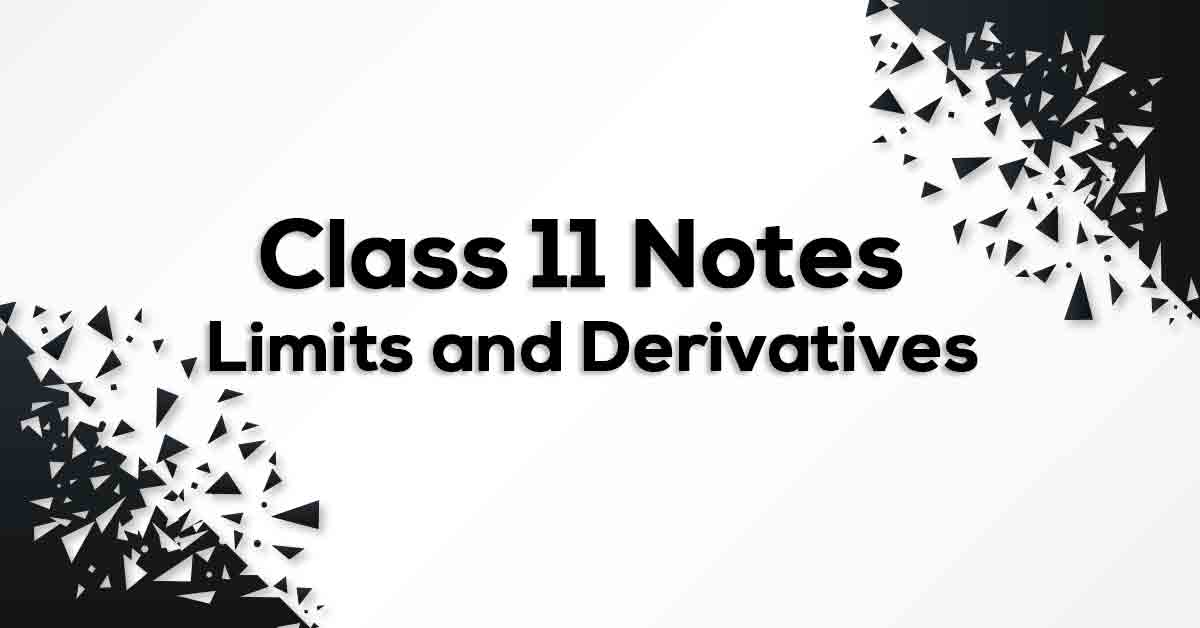Limits and Derivatives Class 11 Formulas & Notes | Vidyakul

# Limits and Derivatives Class 11 Formulas & Notes## Class 11 Math Chapter 13 Limits and Derivatives Formulae - PDF Download

Limits and Derivatives Class 11 Formulas & Notes are cumulated by our panel of highly experienced teachers to provide the students with effective exam preparation. The pdf not only includes the list of formulae but also offer students with the summary of the chapter, important points to remember and detailed explanation of important concepts and derivations for better understanding and retaining of the chapter. Going through these Limits and Derivatives Class 11 Formulas & Notes would easily help students to grasp challenging concepts which would ultimately lead them to score maximum marks in their exams.

### What do you mean by Limits and Derivatives?

Calculus is that branch of mathematics which mainly deals with the study of change in the value of a function as the points in the domain change. Limit is used when we have to find value of a function near to some value. If right & left-hand limits coincide, we call that common value as limit of f(x) at x = a & denote it by lim x→a f(x). The derivative measures the instantaneous rate of change of the function, as distinct from its average rate of change. It is defined as the limit of the average rate of change in the function as the length of the interval on which the average is computed tends to zero.